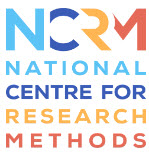Hi, you are logged in as , if you are not , please click here
You are shopping as , if this is not your email, please click here

# Period Cohort Analysis - online

Info

## Course InformationAge, period and cohort (APC) are three ways in which things change over time; however they are exactly collinear, in that if we know an individual’s age and year of measurement, we can work out their birth year (age=period-cohort). This presents problems for any longitudinal analysis, because we cannot include all three APC terms in a statistical model without some kind of constraint. Yet if we fail to include all three terms in a model, we can radically mis-apportion affects: what can, for example, appear to be an age effect, could in fact be a combination of period and cohort effects.

This course will introduce the age-period-cohort identification problem, and what it means for longitudinal social science research. We will consider some supposed solutions to the identification problem, some old (such as the combining age categories) and some new (such as the hierarchical age-period-cohort model) and consider the extent to which these can and cannot produce accurate age-period-cohort estimates. Finally, we will consider what we can do as researchers interested in age, period and cohort effects – both in terms of statistical models, and data visualisation

The course will include practical elements, taught using R.
R and RStudio – students should install these on their computer in advance of the course.

Course Timings: 09:30 – 17:00
Students should have some familiarity with R, since the course will not have time to go over the basics of the software (although code will be provided for students to follow). Students should also have a decent understanding of multiple regression models, and ideally some experience of multilevel models (although we will give a brief introduction to this in the class).

Course Contents:
• The age period cohort identification problem
• Statistical models with various combinations of age, period and cohort, and the assumptions they make
• The problem with statistical “solutions” to the identification problem
• Best practice with APC analysis: statistical models
• Best practice with APC analysis: data visualisation – lexis plots

By the end of this workshop you will:
•    Understand the age period cohort identification problem, and the challenges it raises for longitudinal research
•    Be able to critique supposed solutions to the identification problem, carefully considering the assumptions that those solutions make
•    Develop statistical models that make APC assumptions explicit, based on theory
•    Produce data visualisations (in particular, lexis plots) to show APC patterns in data

#### Course Code

NCRMEDPCA
StartEndPlaces LeftCourse FeeChange Code